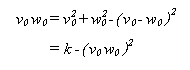Equations

Unless you are following a style guide that specifies otherwise, observe the following conventions.

Integrate equations into the body of your document by including in the text one or more explicit references to each one and, if appropriate, a short explanation of each term.

Include only the main equations in the body of the document. Detailed derivations and calculations, if appropriate, should be put in appendixes.

Set off equations from the text by displaying them, centered on the longest line, and with equation numbers as shown.

An ion in crossed electric and magnetic fields drifts at right angles to both fields with a velocity of(Eq. 7)

where Vd is the drift velocity, Es is the magnitude of the static field, and B is the magnetic field strength. If Vd were to approach unity, the behavior of the electric . . .

Guidelines for Displaying Equations

• Align runover lines on the equals signs (=):
•• Break an equation longer than one line according to the following order of preference:
1. Immediately before the equals sign. Align the equals sign in the second line with the first operator in the first line.
2. Immediately before an addition or subtraction sign (+ or -) that is not enclosed in parentheses, brackets, or braces. Align the addition or subtraction sign starting the second line with the equals sign in the first line.
3. Immediately before a multiplication sign (×). Align the multiplication sign starting the second line with the equals sign in the first line.
4. Between two sets of enclosures (parentheses, brackets, or braces). Begin the second line with an explicit multiplication sign aligned with the equals sign followed by the second enclosed set.## Equations ##